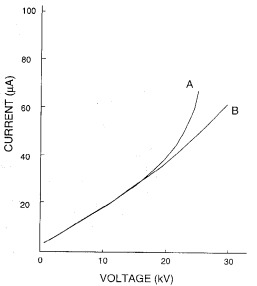• Select the optimal voltage setting for your separation
• Maximize the Efficiency of your method
• Learn the upper Voltage Limits of your method

Performing an Ohm’s Law Plot or Current/Voltage Plot is very simple. This information is essential to understanding the limits of your method, which could come in handy when the method is exported to another lab. The optimal voltage is a function capillary diameter, capillary length, BGE concentration, BGE ionic mobilities, and the temperature of your system and efficiency of your cooling system.

The point at which there is a positive deviation from linearity of this plot is the maximum voltage you should use with the conditions you tested under.

• Fill your capillary with a buffer or BGE that you will use in your SOP.
• Set the instrument temperature to the temperature in your SOP.
• Set the voltage to 1-2kV.
• Activate and record the Current.
• Increase the voltage in 1-5kV increments. Record the current once it stabilizes each time.
• Enter the Data in a spreadsheet program and perform a plot of Current V. Voltage
• Use voltage as the X-axis.
• Determine when a 5% positive deviation from linearity occurs.
This is the OPTIMAL Voltage to run at.

If you change any experimental conditions you should re-run the above Ohm’s Law Plot.

This Ohm’s Law Plot shows the difference between two different instruments with two different cooling systems. Plot A, uses an air-cooled temperature control system and Plot B, uses a liquid cooled system. Capillaries and buffers were the same. It is obvious by this graph that a liquid cooled system is more efficient and provides a greater capability. It does bring in other factors such as cost and is a little less easy to use.